## What is Interest Rate?

The term interest rate is used differently in different contexts. A common usage of interest rate is to determine the amount of interest payable on borrowings such as mortgages or other loans. This is from the perspective of the borrower. However, from the perspective of an investor, the interest rate implies the percentage rate of return on investments. When faced with multiple investment alternatives, it can help in determining the most profitable ones. Interest rate is denoted either by `i’ or `r’ interchangeably.

## Key Learning Points

• The interest rate is cost of financing incurred by a borrower of debt in percentage terms
• From the perspective of the creditor, interest rate is the rate of return they expect to receive on their loan
• Interest rates are used in many areas of finance and are commonly denoted as either “i” or “r”
• Yield is the annual return an investor realizes on an investment
• The internal interest rate or IRR is the discount rate which makes the value of future cash flows equal to the initial investment

## Yields

Interest rate is also referred to as yields, discount rate and annual return. The formula for calculating the interest rate is as follows: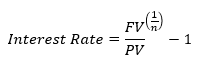Where

FV = Future value

PV = Present value

N = Number of periods

### Example 1

Let us understand this with an example. You are offered an investment proposal to invest 75 now and get 150 after 10 years. What will be the interest rate or annual return on this investment?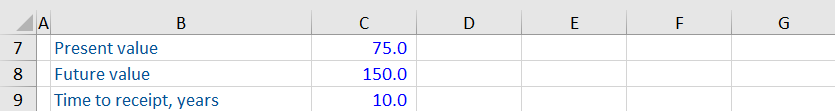Using the formula above, you can get the following as the interest rate or annual return:

Annual return = (150/75)^ (1/10) -1

= 7.2%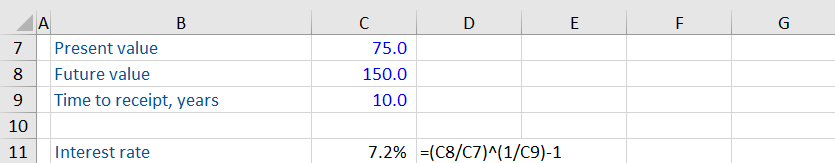## Example 2

Let’s look at another example. You are planning to buy 1 share of Tesla at the current market price of 1,418. A research firm is predicting the stock’s price to reach 1,700 in 3 year’s time. What is the annual return on this investment?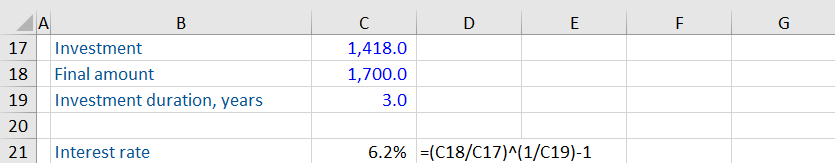## Internal Rate of Return

The formula mentioned above is useful for calculating the interest rate or return on a single stream of cash flows. However, some investments may require multiple streams of cash outflows (or investment by the investor) at different periods and give a single sum in return. In such scenarios, the internal rate of return (IRR) is useful in comparing different investment proposals and choosing one that generates the highest returns.

For example, you are asked to invest 50 for each of the next 4 years and are promised 250 in the fifth year.

You can calculate the return on this investment by using the internal rate of return (IRR) feature in Excel.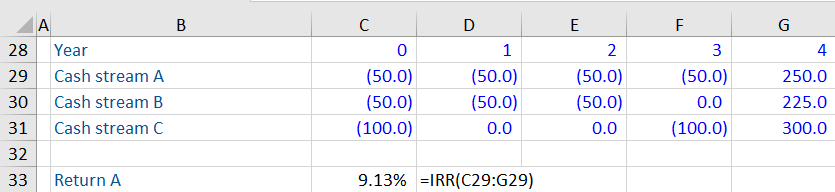Let us compare these returns with another investment proposal.

You have been asked to invest 50 for each of the next 3 years and have been assured a sum of 225 at the end of 5 years.

Using the IRR formula in Excel for this stream of cash flows, we get the returns as follows: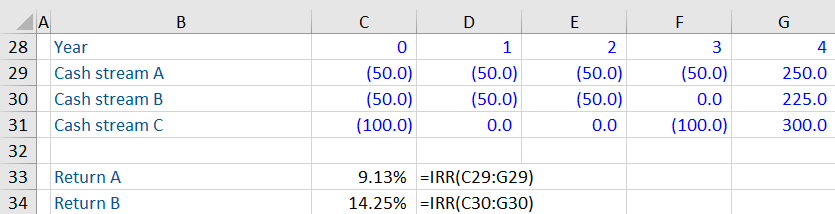Notice the difference here. While proposal A is giving a higher amount (250) than proposal B (225) at the end of the fifth year, proposal B is giving a higher return (14.25%). Given a choice between the two proposals, proposal 2 is more attractive financially.